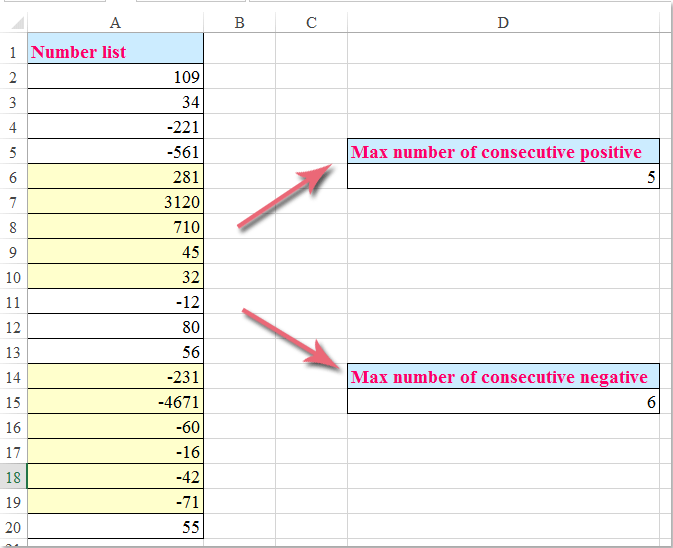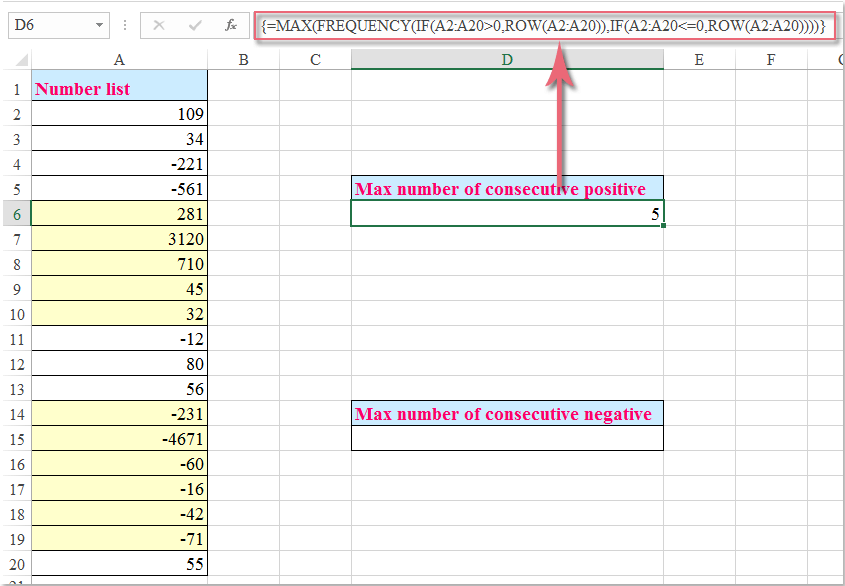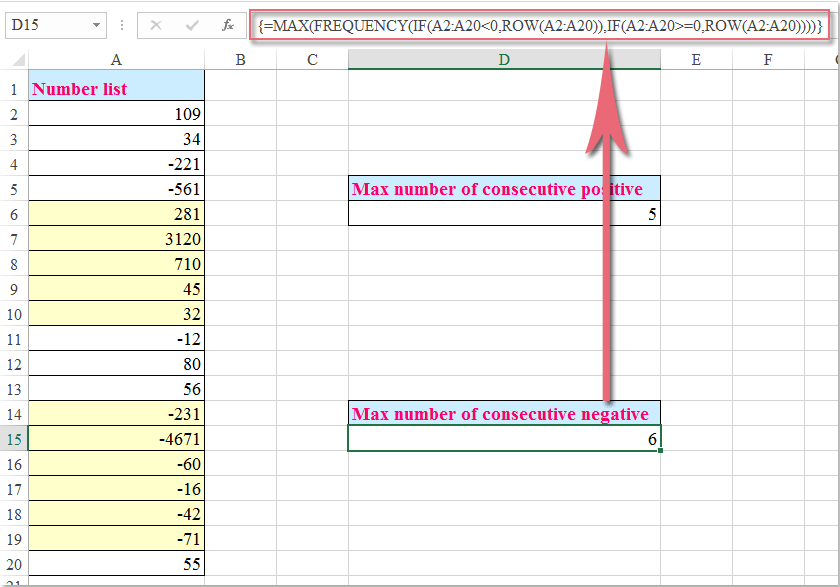## How to count max number of consecutive positive / negative numbers in Excel?

If you have a list of data which mix with positive and negative numbers, and now, you want to count the max number of consecutive positive and negative numbers as following screenshot shown, how could you deal with this task in Excel?Count the max number of consecutive positive and negative numbers with array formulas

#### Count the max number of consecutive positive and negative numbers with array formulas###### Save 50% of your time, and reduce thousands of mouse clicks for you every day!

To get the max number of consecutive positive and negative numbers, please apply the following array formulas:

Count the max number of consecutive positive numbers:

Enter this formula into a cell where you want to get the result:

=MAX(FREQUENCY(IF(A2:A20>0,ROW(A2:A20)),IF(A2:A20<=0,ROW(A2:A20)))), and then press Ctrl + Shift + Enter keys together, and you will get the correct result as you need, see screenshot:Count the max number of consecutive negative numbers:

Enter this formula into a cell where you want to get the result:

=MAX(FREQUENCY(IF(A2:A20<0,ROW(A2:A20)),IF(A2:A20>=0,ROW(A2:A20)))), and then press Ctrl + Shift + Enter keys simultaneously, and you will get the result as you need, see screenshot:Note: In the above formulas, A2:A20 is the list of cell range which you want to use.

### Best Office Productivity Tools

Supercharge Your Spreadsheets： Experience Efficiency Like Never Before with Kutools for Excel

 Popular Features: Find/Highlight/Identify Duplicates   |  Delete Blank Rows   |  Combine Columns or Cells without Losing Data   |   Round without Formula ... Super Lookup: Multiple Criteria VLookup  |   Multiple Value VLookup  |   VLookup Across Multiple Sheets   |   Fuzzy Lookup .... Advanced Drop-down List: Quickly Create Drop Down List   |  Dependent Drop Down List   |  Multi-select Drop Down List .... Column Manager: Add a Specific Number of Columns   |   Move Columns   |   Unhide Columns   |   Compare Columns to Select Same & Different Cells ... Featured Features: Grid Focus   |  Design View   |   Big Formula Bar   |  Workbook & Sheet Manager   |  Resource Library (Auto Text)   |  Date Picker   |  Combine Worksheets   |  Encrypt/Decrypt Cells   |  Send Emails by List   |  Super Filter   |   Special Filter (filter bold/italic/strikethrough...) ... Top 15 Toolset:  12 Text Tools (Add Text, Remove Characters, ...)   |   50+ Chart Types (Gantt Chart, ...)   |   40+ Practical Formulas (Calculate age based on birthday, ...)   |   19 Insertion Tools (Insert QR Code, Insert Picture from Path, ...)   |   12 Conversion Tools (Numbers to Words, Currency Conversion, ...)   |   7 Merge & Split Tools (Advanced Combine Rows, Split Cells, ...)   |   Many More...

Kutools for Excel boasts over 300 features, ensuring that what you need is just a click away...

Supports Office/Excel 2007-2021 & newer, including 365   |   Available in 44 languages   |   Enjoy a full-featured 30-day free trial.#### Office Tab Brings Tabbed interface to Office, and Make Your Work Much Easier

• Enable tabbed editing and reading in Word, Excel, PowerPoint, Publisher, Access, Visio and Project.
• Open and create multiple documents in new tabs of the same window, rather than in new windows.
• Increases your productivity by 50%, and reduces hundreds of mouse clicks for you every day!Rated 5 out of 5 · 1 ratings
This comment was minimized by the moderator on the site
PPPPPPPQQQQPPPPQQPPQQPPPPPP

I have a data like above. I have applied the formula that you have suggested. It was working fine. Answer was 7.

={MAX(FREQUENCY(IF(A4:AA4=P, COLUMN(A4:AA4)),IF(A4:AA4<>P, COLUMN(A4:AA4))))}
But now my requirement is this formula should consider only the last 10 cells from the formula applied cells. and the answer should come as 6.
Is it Possible.
This comment was minimized by the moderator on the site
Hi,
This was very useful. Thank You very much !!
A question, what if in the data array I want to ignore blanks or zeros. Like the if the next number after blank or zero is positive it should add in frequency

This comment was minimized by the moderator on the site
Hello, Naveen
``=MAX(FREQUENCY(IF(A2:A20>=0,ROW(A2:A20)),IF(A2:A20<0,ROW(A2:A20))))``

After copying and pasting the formula, please remember to press Ctrl + Shift + Enter keys together to get the correct result.
This comment was minimized by the moderator on the site
안녕하세요. 감사합니다. 데이터 필터된 상황의 경우에도 연속 손실이나 이익 수를 계산할 수 있는지 궁금합니다. 그리고 데이터 필터된 상횡의 평균 연속손실 수를 계산하는 방법은 있습니까?
Rated 5 out of 5
This comment was minimized by the moderator on the site
Wonderful, thank you! I'm using your formula now, but I have a question: Is there any way to further filter the data so that it only counts consecutive positive/negative cells IF they share a value in a different column? For example, if, using your example above, in Column B (next to your Number List in Column A) the cells were filled with either the letter 'A' or 'B' in random order, what formula would you use to determine the max number of positive cells that are ALSO consecutive A's in Column B?
This comment was minimized by the moderator on the site
It's amazing, just wondering if there a way to highlight consecutives?
This comment was minimized by the moderator on the site
I get an #Value output if there are only a streak of positive numbers only. Also, I get the same error, if there is only negative streak of numbers. It works fine when there are at least 1 positive and at least 1 negative number on the column. Does anybody knows how to fix this issue. I am using open office.Which uses the same commands, except for the " ; " instead of " , " as a separator.
This comment was minimized by the moderator on the site
Hello, Pablo,
The formulas work well in my wotksheet when there are a list of positive or negative numbers, do you press Ctrl + Shift + Enter keys together to get the result?
This comment was minimized by the moderator on the site
Hello,
Tank you for this post.I'm trying to get the average number of consecutive negative number from a row.I've been trying to do it myself but I can't figure it out.
Would you mind helping me ?
Thanks !
This comment was minimized by the moderator on the site
So, usefull context. I was jus making a video for my Youtube Channel. When i googeled some key word, i came across with your formula. First of all, thank you. And secondly i will give reference to this website for the formulas explanation. I have not so many subscriber, but it may give your website a littele bit more traffic. Thank you and have nice day.
This comment was minimized by the moderator on the site
Hi, thank you very much for the information! It's very useful :) I wondering if is posible to add up the Max Number of consecutive negatives? I will apreciate a lot if you can help me. Thank you!!
This comment was minimized by the moderator on the site
Hi, Joan,

Sum max number of consecutive positive numbers: (Note: D2 is the cell of the consecutive positive numbers you have counted by the using the formula in this article, please press Ctrl + Shift + Enter keys together)
=MAX((COUNTIF(OFFSET(A2:A18,ROW(A2:A18)-ROW(A2),0,D2),">=0")=D2)*SUBTOTAL(9,OFFSET(\$A\$2:\$A\$18,ROW(A2:A18)-ROW(\$A\$2),0,D2)))

Sum max number of consecutive negative numbers: (Note: E2 is the cell of the consecutive negative numbers you have counted by the using the formula in this article, please press Ctrl + Shift + Enter keys together)
=MIN((COUNTIF(OFFSET(A2:A18,ROW(A2:A18)-ROW(A2),0,E2),"<0")=E2)*SUBTOTAL(9,OFFSET(\$A\$2:\$A\$18,ROW(A2:A18)-ROW(\$A\$2),0,E2)))

This comment was minimized by the moderator on the site
how do you count consecutive occurrences of two different values or numbers
This comment was minimized by the moderator on the site
How do you do this with multiple rows? say A1:A10 and C1:C10 ???
This comment was minimized by the moderator on the site
Hello, Ryan,
Sorry for that, this formula is only applied to single column or row.
If you have other good ideals, please comment here.
Thank you!
This comment was minimized by the moderator on the site
Not working! Gives the max number of positive results not consecutive ones
This comment was minimized by the moderator on the site
Hi, Ivan,
The above formula only can help you to count the max number of the positive or negative numbers, it can't extract the consecutive cells.
If you have the good ways, please comment here.
Thank you！
This comment was minimized by the moderator on the site
Excelente! Funcionou perfeitamente. E se quisermos somar os números de cada intervalo, como ficaria?
This comment was minimized by the moderator on the site
I want the consecutive positive numbers from last cell of one column in which data is entered. I will have many column i.e. A,B,C,D,E,F etc
This comment was minimized by the moderator on the site
I want the consecutive positive numbers from last of one column. I will have many column i.e. A,B,C,D,E,F etc
There are no comments posted here yet